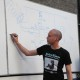## Another Julia Set animation

Click this image to view the Julia Set animation (the gif file is about 5.3MB in size, so I didn’t want to embed it here directly):This is the Python code I used to generate the 200 individual frames in the animation:

```#
# julia_frames.py - Generates Julia Set images
# Written by Ted Burke
# Last updated 10-2-2012
#
# This program generates a sequence of Julia Set images,
# using values of the complex parameter c that lie on a
# circle of radius 0.3, centred on the point -1 + 0j in
# the complex plane. I'm hoping this circular path will
# generate plenty of interesting images since it lies a
# little outside the perimeter of a roughly circular
# lobe (with radius 0.25) of the Mandelbrot Set.
#

import numpy
import math

# Specify image width and height
w, h = 640, 480

# Specify real and imaginary range of image
re_min, re_max = -2.0, 2.0
im_min, im_max = -1.5, 1.5

# Generate evenly spaced values over real and imaginary ranges
real_range = numpy.arange(re_min, re_max, (re_max - re_min) / w)
imag_range = numpy.arange(im_max, im_min, (im_min - im_max) / h)

# Frame counter
frame = 0

# Iterate over a range of c values
for angle in numpy.arange(0.0, 2 * math.pi, 0.01 * math.pi):
# Increment frame counter
frame += 1

# Open file and write PGM header info
filename = "{0:03d}.pgm".format(frame)
print filename
fout = open(filename, 'w')
fout.write('P2\n# Julia Set image\n' + str(w) + ' ' + str(h) + '\n255\n')

# Generate pixel values
for im in imag_range:
for re in real_range:
z = complex(re, im)
c = complex(-1.0 + 0.3*math.sin(angle),0.3*math.cos(angle))
n = 255
while abs(z) < 10 and n >= 5:
z = z*z + c
n = n - 5
# Write pixel value to file
fout.write(str(n) + ' ')
fout.write('\n')

# Close file
fout.close()
```

To convert each of the 200 images from PGM to PNG format, I used the following command:

```for i in {001..200}; do convert \$i.pgm \$i.png; done
```

To combine the 200 PNG images into a single animated gif, I used the following command:

```convert -delay 10 *.png julia.gif
```
This entry was posted in Uncategorized and tagged , , , , , , , , , . Bookmark the permalink.

### 3 Responses to Another Julia Set animation

1.Joe Yuan says:

Cool stuff! Trying to replicate this in Processing 2.0

•batchloaf says:

Interesting! I hope it works out. I’d be interested to see what it looks like in processing. Do you think you’ll put it online?

Ted

2.John Kennedy Peña says:

uhh, i’m a noob, where can i edit the colors?